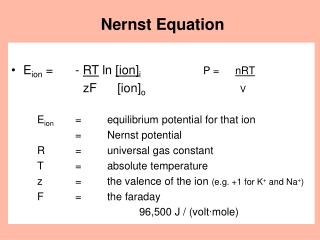Download PresentationNernst EquationNernst Equation - PowerPoint PPT Presentation

Download PresentationNernst Equation
An Image/Link below is provided (as is) to download presentation

Download Policy: Content on the Website is provided to you AS IS for your information and personal use and may not be sold / licensed / shared on other websites without getting consent from its author. While downloading, if for some reason you are not able to download a presentation, the publisher may have deleted the file from their server.

- - - - - - - - - - - - - - - - - - - - - - - - - - - E N D - - - - - - - - - - - - - - - - - - - - - - - - - - -
Presentation Transcript

1. Nernst Equation • Eion = - RT ln [ion]iP = nRT zF [ion]o V Eion = equilibrium potential for that ion = Nernst potential R = universal gas constant T = absolute temperature z = the valence of the ion (e.g. +1 for K+ and Na+) F = the faraday 96,500 J / (volt∙mole)

2. Eion = - RT ln [ion]izF [ion]o • Simplifying the Nernst equation for millivolts, log10, and 37°C yields: Eion (in mV) = +61.5 log [ion]o [for + ions] [ion]i Eion (in mV) = -61.5 log [ion]o [for - ions] [ion]i MEMORIZE

3. see Table 1-2 Ganong Typical Human Neuron in Na+ 15 mM K+ 150 mM voltage -70 mV out 150 mM 5.5 mM 0 mV At the resting membrane potential (-70 mV), is either Na+ or K+ at electrochemical equilibrium?

4. Typical Human Neuron in Na+ 15 mM voltage -70 mV out 150 mM 0 mV concentration gradient electrical gradient Na+ is not at electrochemical equilibrium.

5. ENa • ENa = +61.5 log (150/15) mV = +61.5 log (10) mV = +61.5 (1) mV = +61.5 mV ENa +60 mV MEMORIZE Since -70 mV  +60 mV, Na+ is not in electrochemical equilibrium. What, then, is the electrochemical gradient for Na+?

6. Electrochemical Gradient • electrochemical gradient = measured membrane potential - Eion • for Na+ in a resting neuron: electrochemical gradient = -70 mV - (+60 mV) = - 130 mV • In a resting neuron Na+ is far from equilibrium.

7. Typical Human Neuron in K+ 150 mM voltage -70 mV out 5.5 mM 0 mV concentration gradient electrical gradient Is K+ at electrochemical equilibrium?

8. EK • EK = +61.5 log (5.5/150) mV = +61.5 log (0.037) mV = +61.5 (-1.43) mV = -88 mV EK -90 mV MEMORIZE Since -70 mV  -90 mV, K+ is not in electrochemical equilibrium. What, then, is the electrochemical gradient for K+?

9. Electrochemical Gradient • electrochemical gradient = measured membrane potential - Eion • for K+ in a resting neuron: electrochemical gradient = -70 mV - (-90 mV) = + 20 mV • In a resting neuron, K+ is not in equilibrium, • but it is closer to equilibrium than Na+ is. • To change the membrane potential from -70 to -90 mV, which way would K+ move?

10. Summary outward gradient = 0 mV — inward ENa +60 mV — at rest: Na+ gradient = -130 mV 0 mV — resting membrane potential (neuron) -70 mV — outward gradient = 0 mV — inward at rest: K+ gradient = + 20 mV EK -90 mV — electrical potential of membrane electrochemical gradients for Na+ for K+

11. Goldman (-Hodgkin-Katz) Equation • Neither Na+ nor K+ is in electrochemical equilibrium. • The resting membrane potential is in between the sodium and potassium equilibrium potentials, but closer to the potassium equilibrium potential. • At rest, the permeability of the membrane to potassium is 50-100 x greater than to sodium. • Is the resting membrane potential due to a “tug of war” between K+ and Na+, a “tug of war” in which K+ has the advantage of greater permeability?

12. Goldman (-Hodgkin-Katz) Equation • Goldman, Hodgkin, and Katz modified the Nernst equation to take into account multiple ions and their permeabilities. • Looking at sodium and potassium only: Vrest = 61.5 log PK[K]out + PNa[Na]out(P = permeability) PK[K]in + PNa[Na]in Vrest = - 67 mV • Therefore, passive movements of sodium and potassium account for -67 of the -70 mV of the resting membrane potential.